# Angle (mathematics)

In geometry and trigonometry, an angle (or plane angle) is the figure formed by two rays sharing a common endpoint. The endpoint is called the vertex of the angle. The magnitude of the angle is the "amount of rotation" that separates the two rays, and can be measured by considering the length of circular arc swept out when one ray is rotated about the vertex to coincide with the other (see "Measuring angles," below).

The word angle comes from the Latin word angulus, meaning "a corner." The word angulus is a diminutive, of which the primitive form, angus, does not occur in Latin. Cognate words are the Latin angere, meaning "to compress into a bend" or "to strangle," and the Greek ἀγκύλος (ankylοs), meaning "crooked, curved"; both are connected with the PIE root *ank-, meaning "to bend" or "bow."

## History

Euclid defines a plane angle as the inclination to each other, in a plane, of two lines which meet each other, and do not lie straight with respect to each other. According to Proclus an angle must be either a quality or a quantity, or a relationship. The first concept was used by Eudemus, who regarded an angle as a deviation from a straight line; the second by Carpus of Antioch, who regarded it as the interval or space between the intersecting lines; Euclid adopted the third concept, although his definitions of right, acute, and obtuse angles.

## Measuring angles

In order to measure an angle θ, a circular arc centered at the vertex of the angle is drawn, e.g., with a pair of compasses. The length of the arc s is then divided by the radius of the circle r, and possibly multiplied by a scaling constant k (which depends on the units of measurement that are chosen):$\theta = \frac{s}{r}(k)$

The value of θ thus defined is independent of the size of the circle: if the length of the radius is changed then the arc length changes in the same proportion, so the ratio s/r is unaltered.

In many geometrical situations, angles that differ by an exact multiple of a full circle are effectively equivalent (it makes no difference how many times a line is rotated through a full circle because it always ends up in the same place). However, this is not always the case. For example, when tracing a curve such as a spiral using polar coordinates, an extra full turn gives rise to a quite different point on the curve.

### Units

Angles are considered dimensionless, since they are defined as the ratio of lengths. There are, however, several units used to measure angles, depending on the choice of the constant k in the formula above.

With the notable exception of the radian, most units of angular measurement are defined such that one full circle (i.e. one revolution) is equal to n units, for some whole number n (for example, in the case of degrees, n = 360). This is equivalent to setting k = n/2π in the formula above. (To see why, note that one full circle corresponds to an arc equal in length to the circle's circumference, which is 2πr, so s = 2πr. Substituting, we get θ = ks/r = 2πk. But if one complete circle is to have a numerical angular value of n, then we need θ = n. This is achieved by setting k = n/2π.)

• The degree, denoted by a small superscript circle (°) is 1/360 of a full circle, so one full circle is 360°. One advantage of this old sexagesimal subunit is that many angles common in simple geometry are measured as a whole number of degrees. (The problem of having all "interesting" angles measured as whole numbers is of course insolvable.) Fractions of a degree may be written in normal decimal notation (e.g., 3.5° for three and a half degrees), but the following sexagesimal subunits of the "degree-minute-second" system are also in use, especially for geographical coordinates and in astronomy and ballistics:
• The minute of arc (or MOA, arcminute, or just minute) is 1/60 of a degree. It is denoted by a single prime ( ′ ). For example, 3° 30′ is equal to 3 + 30/60 degrees, or 3.5 degrees. A mixed format with decimal fractions is also sometimes used, e.g., 3° 5.72′ = 3 + 5.72/60 degrees. A nautical mile was historically defined as a minute of arc along a great circle of the Earth.
• The second of arc (or arcsecond, or just second) is 1/60 of a minute of arc and 1/3600 of a degree. It is denoted by a double prime ( ″ ). For example, 3° 7′ 30″ is equal to 3 + 7/60 + 30/3600 degrees, or 3.125 degrees.
• The mil is approximately equal to a milliradian. There are several definitions.
• The full circle (or revolution, rotation, full turn or cycle) is one complete revolution. The revolution and rotation are abbreviated rev and rot, respectively, but just r in rpm (revolutions per minute). 1 full circle = 360° = 2π rad = 400 gon = 4 right angles.
• The right angle is 1/4 of a full circle. It is the unit used in Euclid's Elements. 1 right angle = 90° = π/2 rad = 100 gon.
• The angle of the equilateral triangle is 1/6 of a full circle. It was the unit used by the Babylonians, and is especially easy to construct with ruler and compasses. The degree, minute of arc and second of arc are sexagesimal subunits of the Babylonian unit. One Babylonian unit = 60° = π/3 rad ≈ 1.047197551 rad.
• The grad, also called grade, gradian, or gon is 1/400 of a full circle, so one full circle is 400 grads and a right angle is 100 grads. It is a decimal subunit of the right angle. A kilometer was historically defined as a centi-gon of arc along a great circle of the Earth, so the kilometer is the decimal analog to the sexagesimal nautical mile. The gon is used mostly in triangulation.
• The point, used in navigation, is 1/32 of a full circle. It is a binary subunit of the full circle. Naming all 32 points on a compass rose is called "boxing the compass." 1 point = 1/8 of a right angle = 11.25° = 12.5 gon.
• The astronomical hour angle is 1/24 of a full circle. The sexagesimal subunits were called minute of time and second of time (even though they are units of angle). 1 hour = 15° = π/12 rad = 1/6 right angle ≈ 16.667 gon.
• The binary degree, also known as the binary radian (or brad), is 1/256 of a full circle. The binary degree is used in computing so that an angle can be efficiently represented in a single byte.
• The grade of a slope, or gradient, is not truly an angle measure (unless it is explicitly given in degrees, as is occasionally the case). Instead it is equal to the tangent of the angle, or sometimes the sine. Gradients are often expressed as a percentage. For the usual small values encountered (less than 5%), the grade of a slope is approximately the measure of an angle in radians.

### Positive and negative angles

A convention universally adopted in mathematical writing is that angles given a sign are positive angles if measured counterclockwise, and negative angles if measured clockwise, from a given line. If no line is specified, it can be assumed to be the x-axis in the Cartesian plane. In many geometrical situations a negative angle of −θ is effectively equivalent to a positive angle of "one full rotation less θ." For example, a clockwise rotation of 45° (that is, an angle of −45°) is often effectively equivalent to a counterclockwise rotation of 360° − 45° (that is, an angle of 315°).

In three dimensional geometry, "clockwise" and "counterclockwise" have no absolute meaning, so the direction of positive and negative angles must be defined relative to some reference, which is typically a vector passing through the angle's vertex and perpendicular to the plane in which the rays of the angle lie.

In navigation, bearings are measured from north, increasing clockwise, so a bearing of 45 degrees is north-east. Negative bearings are not used in navigation, so north-west is 315 degrees.

### Approximations

• 1° is approximately the width of a pinky finger at arm's length
• 10° is approximately the width of a closed fist at arm's length.
• 20° is approximately the width of a handspan at arm's length.

## Types of angleAcute (a), obtuse (b), and straight (c) angles. Here, a and b are supplementary angles.The complementary angles a and b (b is the complement of a, and a is the complement of b).
• An angle of 90° (π/2 radians, or one-quarter of the full circle) is called a right angle.
Two lines that form a right angle are said to be perpendicular or orthogonal.
• Angles smaller than a right angle (less than 90°) are called acute angles ("acute" meaning "sharp").
• Angles larger than a right angle and smaller than two right angles (between 90° and 180°) are called obtuse angles ("obtuse" meaning "blunt").
• Angles equal to two right angles (180°) are called straight angles.
• Angles larger than two right angles but less than a full circle (between 180° and 360°) are called reflex angles.
• Angles that have the same measure are said to be congruent.
• Two angles opposite each other, formed by two intersecting straight lines that form an "X" like shape, are called vertical angles or opposite angles. These angles are congruent.
• Angles that share a common vertex and edge but do not share any interior points are called adjacent angles.
• Two angles that sum to one right angle (90°) are called complementary angles.
The difference between an angle and a right angle is termed the complement of the angle.
• Two angles that sum to a straight angle (180°) are called supplementary angles.
The difference between an angle and a straight angle is termed the supplement of the angle.
• Two angles that sum to one full circle (360°) are called explementary angles or conjugate angles.
• The smaller angle at a point where two line segments join is called the interior angle.
In Euclidean geometry, the measures of the interior angles of a triangle add up to π radians, or 180°; the measures of the interior angles of a simple quadrilateral add up to 2π radians, or 360°. In general, the measures of the interior angles of a simple polygon with n sides add up to [(n − 2) × π] radians, or [(n − 2) × 180]°.
• The angle supplementary to the interior angle is called the exterior angle.
• The angle between two planes (such as two adjacent faces of a polyhedron) is called a dihedral angle. It may be defined as the acute angle between two lines normal to the planes.
• The angle between a plane and an intersecting straight line is equal to ninety degrees minus the angle between the intersecting line and the line that goes through the point of intersection and is normal to the plane.
• If a straight transversal line intersects two parallel lines, corresponding (alternate) angles at the two points of intersection are congruent; adjacent angles are supplementary (that is, their measures add to π radians, or 180°).

## A formal definition

### Using trigonometric functions

A Euclidean angle is completely determined by the corresponding right triangle. In particular, if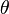$\theta$ is a Euclidean angle, it is true that$\cos \theta = \frac{x}{\sqrt{x^2 + y^2}}$

and$\sin \theta = \frac{y}{\sqrt{x^2 + y^2}}$

for two numbers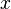$x$ and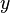$y$. So an angle in the Euclidean plane can be legitimately given by two numbers$x$ and$y$.

To the ratio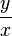$\frac{y}{x}$ there correspond two angles in the geometric range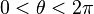$0 < \theta < 2\pi$, since$\frac{\sin \theta }{\cos \theta } = \frac{\frac{y}{\sqrt{x^2 + y^2}}}{\frac{x}{\sqrt{x^2 + y^2}}} = \frac{y}{x} = \frac{-y}{-x} = \frac{\sin (\theta + \pi)}{\cos (\theta + \pi) }$

### Using rotations

Suppose we have two unit vectors$\vec{u}$ and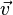$\vec{v}$ in the euclidean plane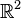$\mathbb{R}^2$. Then there exists one positive isometry (a rotation), and one only, from$\mathbb{R}^2$ to$\mathbb{R}^2$ that maps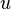$u$ onto$v$. Let r be such a rotation. Then the relation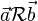$\vec{a}\mathcal{R}\vec{b}$ defined by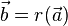$\vec{b}=r(\vec{a})$ is an equivalence relation and we call angle of the rotation r the equivalence class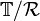$\mathbb{T}/\mathcal{R}$, where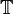$\mathbb{T}$ denotes the unit circle of$\mathbb{R}^2$. The angle between two vectors will simply be the angle of the rotation that maps one onto the other. We have no numerical way of determining an angle yet. To do this, we choose the vector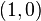$(1,0)$, then for any point M on$\mathbb{T}$ at distance$\theta$ from$(1,0)$ (on the circle), let$\vec{u}=\overrightarrow{OM}$. If we call$r_\theta$ the rotation that transforms$(1,0)$ into$\vec{u}$, then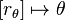$\left[r_\theta\right]\mapsto\theta$ is a bijection, which means we can identify any angle with a number between 0 and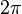$2\pi$.

## Angles between curves

The angle between a line and a curve (mixed angle) or between two intersecting curves (curvilinear angle) is defined to be the angle between the tangents at the point of intersection. Various names (now rarely, if ever, used) have been given to particular cases:—amphicyrtic (Gr. ἀμφί, on both sides, κυρτόσ, convex) or cissoidal (Gr. κισσόσ, ivy), biconvex; xystroidal or sistroidal (Gr. ξυστρίσ, a tool for scraping), concavo-convex; amphicoelic (Gr. κοίλη, a hollow) or angulus lunularis, biconcave.

## The dot product and generalisation

In the Euclidean plane, the angle θ between two vectors u and v is related to their dot product and their lengths by the formula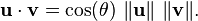$\mathbf{u} \cdot \mathbf{v} = \cos(\theta)\ \|\mathbf{u}\|\ \|\mathbf{v}\|.$

This allows one to define angles in any real inner product space, replacing the Euclidean dot product · by the Hilbert space inner product <·,·>.

## Angles in Riemannian geometry

In Riemannian geometry, the metric tensor is used to define the angle between two tangents. Where U and V are tangent vectors and gij are the components of the metric tensor G,$\cos \theta = \frac{g_{ij}U^iV^j} {\sqrt{ \left| g_{ij}U^iU^j \right| \left| g_{ij}V^iV^j \right|}}.$

## Angles in geography and astronomy

In geography we specify the location of any point on the Earth using a Geographic coordinate system. This system specifies the latitude and longitude of any location, in terms of angles subtended at the centre of the Earth, using the equator and (usually) the Greenwich meridian as references.

In astronomy, we similarly specify a given point on the celestial sphere using any of several Astronomical coordinate systems, where the references vary according to the particular system.

Astronomers can also measure the angular separation of two stars by imagining two lines through the centre of the Earth, each intersecting one of the stars. The angle between those lines can be measured, and is the angular separation between the two stars.

Astronomers also measure the apparent size of objects. For example, the full moon has an angular measurement of approximately 0.5°, when viewed from Earth. One could say, "The Moon subtends an angle of half a degree." The small-angle formula can be used to convert such an angular measurement into a distance/size ratio.